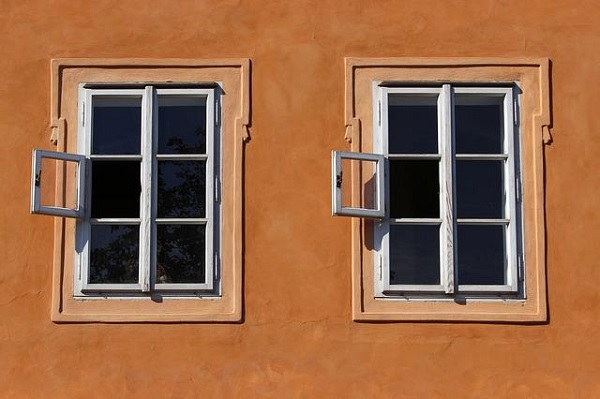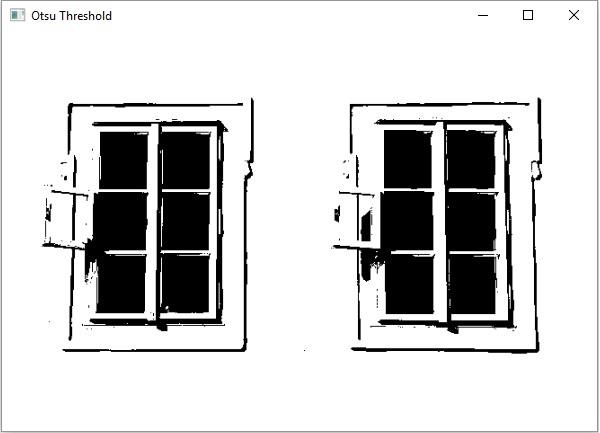# Explain Otsu threshold technique in OpenCV using a Java Example

JavaObject Oriented ProgrammingProgramming

Thresholding is a simple technique for the segmentation of an image. it is often used to create binary images. In this the pixels greater than a given threshold value will be replaced with a standard value

• In simple, the threshold value is constant throughout the image.

• Adaptive thresholding the threshold value is calculated for smaller regions and therefore, there will be different threshold values for different regions.

Whereas in the Otsu threshold technique the threshold value is determined automatically, it chooses an optimal threshold value based on the image histogram.

The threshold() method of the Imgproc class accepts

• Two Mat objects representing the source and destination images.

• Two integer variables representing the threshold or the standard value.

• An integer variable representing the type of the simple threshold.

As parameters and performs the threshold operation on the given image and stores the result in the destination matrix.

To the Otsu threshold technique on an image invoke this method bypassing the Imgproc.THRESH_OTSU as the type of the technique.

## Example

import java.awt.Image;
import java.awt.image.BufferedImage;
import java.io.IOException;
import javafx.application.Application;
import javafx.embed.swing.SwingFXUtils;
import javafx.scene.Group;
import javafx.scene.Scene;
import javafx.scene.image.ImageView;
import javafx.scene.image.WritableImage;
import javafx.stage.Stage;
import org.opencv.core.Core;
import org.opencv.core.Mat;
import org.opencv.highgui.HighGui;
import org.opencv.imgcodecs.Imgcodecs;
import org.opencv.imgproc.Imgproc;
public class OtsuThresholding extends Application {
public void start(Stage stage) throws IOException {
String file ="D:\\Images\\win2.jpg";
//Creating an empty matrices to store the destination image.
Mat dst = new Mat(src.rows(), src.cols(), src.type());
//Applying simple threshold
Imgproc.threshold(src, dst, 50, 255, Imgproc.THRESH_OTSU);
//Converting matrix to JavaFX writable image
Image img = HighGui.toBufferedImage(dst);
WritableImage writableImage= SwingFXUtils.toFXImage((BufferedImage) img, null);
//Setting the image view
ImageView imageView = new ImageView(writableImage);
imageView.setX(10);
imageView.setY(10);
imageView.setFitWidth(575);
imageView.setPreserveRatio(true);
//Setting the Scene object
Group root = new Group(imageView);
Scene scene = new Scene(root, 595, 400);
stage.setTitle("Otsu Threshold");
stage.setScene(scene);
stage.show();
}
public static void main(String args[]) {
launch(args);
}
}

## Input Image## Output